# Plot Run Time Data

Use the Plot tool to track converged and derived solution behavior in real time.

## Open .log Files and View Plots

1. Open a .log file in the Plot tool in the following ways:
• From the Solution ribbon, click the Plot tool.

In the Plot Utility dialog, click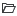then browse and select a file.

• From the Run Status dialog, right-click on an AcuSolve run and select Plot time history from the context menu.
2. Double-click on an option in the library to view the plot in a new tab.
Convergence plots for residual ratios and solution ratios are available by default.

If AcuSolve is still running, the plots update in real time to track the solution progress.

Tip:
• Left-click and draw a window in the plot to zoom in on the defined area. Right-click to return to the default view.
• Click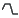to change the scale from linear to logarithmic.

## Create Plots

1. From the Solution ribbon, click the Plot tool.
The Plot Utility dialog opens.
2. Click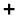in the tab area to create a new plot.
3. Enter a title for the plot.
4. Select an x-axis parameter.
5. Define the y-axis.
1. Select a data type.
Run Data
Contains the general simulation parameters such as Time, Time Increment, CPU Time and Elapsed Time.
Residual ratio
Contains the residual ratios for each of the flow parameters such as pressure, velocity, eddy viscosity and temperature. These ratios can be plotted with three different options Initial, Final and All.
Solution ratio
Contains the solution ratios for each of the flow parameters such as pressure, velocity, eddy viscosity and temperature. These ratios can be plotted with three different options Initial, Final and All.
Surface output
Contains surface integrated values of variables such as pressure, momentum, mass flux and wall shear stress.
Surface statistics
Contains the surface statistics of minimum and maximum of variables such as pressure, momentum, mass flux and wall shear stress.
2. For residual and solution ratios, select a residual type.
Initial
Provides the ratios at the start of each time step.
Final
Provides the ratios at the end of each time step.
All
Provides the ratios for all of the staggers within a time step.
3. For surface output and statistics, select a variable sub-category.
Direction orientated variables, such as velocity, momentum, and shear stress require you to select a coordinate direction. Clickbelow the y-axis definition to plot multiple directions.
4. Activate the check boxes for the surfaces or variables you want to plot.
5. Optional: Click the color patch next to a variable/surface, then use the Select Color dialog to change the plot color.
Tip: Activate Twin Y-Axis to define a second set of y-axis variables.
6. Click Apply.
The plot is added to the library.

## Edit Plots

1. From the Solution ribbon, click the Plot tool.
The Plot Utility dialog opens.
2. From either the plot library or an individual plot tab, click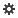.
3. Edit the name, axis values, and colors then click Apply.

## Delete Plots

1. From the Solution ribbon, click the Plot tool.
The Plot Utility dialog opens.
2. Open a plot.
3. Click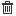.

## Export Plots

1. From the Solution ribbon, click the Plot tool.
The Plot Utility dialog opens.
2. From the plot library, click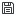to save the entire plot workspace.
3. From a plot tab, click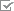then select Export to save the individual plot.